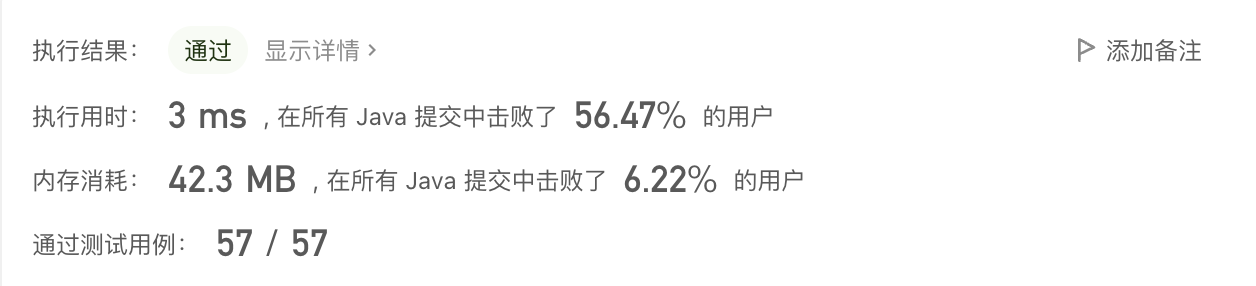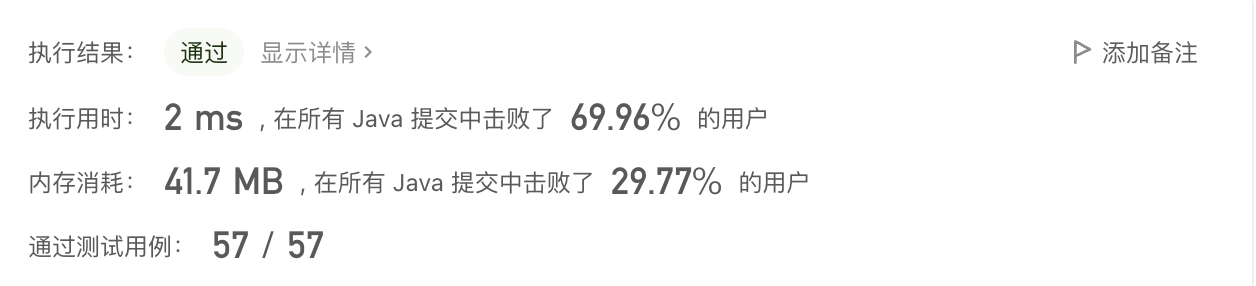# 【萌新解题】两数之和

• 2022 年 7 月 13 日
• 本文字数：2753 字

阅读完需：约 9 分钟class Solution {    public int[] twoSum(int[] nums, int target) {        int len = nums.length;        for (int i=0; i<len; ++i) {            for (int j=i+1; j<len; ++j) {                if (nums[i] + nums[j] == target) {                    return new int[]{i, j};                }            }        }        return new int[]{-1, -1};    }}

class Solution {    public int[] twoSum(int[] nums, int target) {        // 哈希表的 key 是 nums[i]，value 是 i        Map<Integer, Integer> cache = new HashMap<>();        // 输入数组长度        int len = nums.length;        // 初始化哈希表        for (int i=0; i<len; ++i) {            cache.put(nums[i], i);        }        for (int i=0; i<len; ++i) {            // 两数之和 sum 转换成首先固定一个数 A，那另外一个数就是 another = sum-A            int another = target - nums[i];            // 如果 another 也在哈希表中，且不是 nums[i] 时，说明找到两数之和的另外一个数            if (cache.containsKey(another) && i != cache.get(another)) {                return new int[]{i, cache.get(another)};            }        }        // 没有找到符合条件的两个数，我们返回异常值即可        return new int[]{-1, -1};    }}for (int i=0; i<len; ++i) {    // 初始化哈希表    cache.put(nums[i], i);    // 两数之和 sum 转换成首先固定一个数 A，那另外一个数就是 another=sum-A    int another = target - nums[i];    // 如果 another 也在哈希表中，且不是 nums[i] 时，说明找到两数之和的另外一个数    if (cache.containsKey(another) && i != cache.get(another)) {        return new int[]{i, cache.get(another)};    }}

class Solution {    public int[] twoSum(int[] nums, int target) {        // 哈希表的 key 是 nums[i]，value 是 i        Map<Integer, Integer> cache = new HashMap<>();        // 输入数组长度        int len = nums.length;        for (int i=0; i<len; ++i) {            // 两数之和 sum 转换成首先固定一个数 A，那另外一个数就是 another=sum-A            int another = target - nums[i];            // 如果 another 也在哈希表中，且不是 nums[i] 时，说明找到两数之和的另外一个数            if (cache.containsKey(another) && i != cache.get(another)) {                return new int[]{i, cache.get(another)};            }            // 初始化哈希表            cache.put(nums[i], i);        }        // 没有找到符合条件的两个数，我们返回异常值即可        return new int[]{-1, -1};    }}class Solution {    public int[] twoSum(int[] nums, int target) {        // 先将数组排好序        Arrays.sort(nums);        // 算出数组长度        int len = nums.length;        // 左指针指向数组开头，并逐步往中间移动        int left = 0;        // 右指针指向数组末尾，并逐步往中间移动        int right = len - 1;        // 开始循环        while (left < right) {            int sum = nums[left] + nums[right];            if (target == sum) {                // 找到后，返回两数在数组中的下标                return new int[]{left, right};            } else if (target < sum) {                // 当两数之和大于目标值时，由于数组已经从小到大排序，因此，右指针要往中间移动                right--;            } else if (target > sum) {                // 当两数之和小于目标值，由于数组已经从小到大排序，因此，左指针要往中间移动                left++;            }        }        return new int[]{-1, -1};    }}## 评论